## del Pezzo surfaces

Here are the G-functions (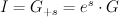$I = G_{+s} = e^s \cdot G$)
and regularized quantum periods (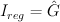$I_{reg} = \hat{G}$)
for del Pezzo surfaces.

### The del Pezzo surface of degree 9

This is the toric variety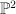$\PP^2$.  We have: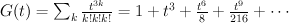$G(t) = \sum_k {t^{3k} \over k!k!k!} = 1+t^3+\frac{t^6}{8}+\frac{t^9}{216}+\cdots$
and the regularized quantum period is: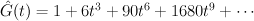$\hat{G}(t) = 1+6 t^3+90 t^6+1680 t^9+\cdots$

### The del Pezzo surface of degree 8, case a

This is the toric variety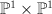$\PP^1 \times \PP^1$. We have: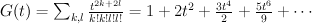$G(t) = \sum_{k,l} {t^{2k+2l} \over k!k!l!l!} = 1+2 t^2+\frac{3 t^4}{2}+\frac{5 t^6}{9}+\cdots$
and the regularized quantum period is: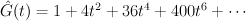$\hat{G}(t) = 1+4 t^2+36 t^4+400 t^6+\cdots$

### The del Pezzo surface of degree 8, case b

This is the toric variety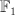$\mathbb{F}_1$. We have: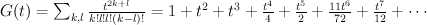$G(t) = \sum_{k,l} {t^{2k+l} \over k!l!l!(k-l)!} = 1+t^2+t^3+\frac{t^4}{4}+\frac{t^5}{2}+\frac{11 t^6}{72}+\frac{t^7}{12}+\cdots$
and the regularized quantum period is: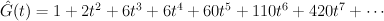$\hat{G}(t) = 1+2 t^2+6 t^3+6 t^4+60 t^5+110 t^6+420 t^7+\cdots$

### The del Pezzo surface of degree 7

This is the blow-up of$\PP^2$ in two points. It is a toric variety. We have: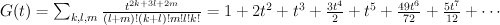$G(t) = \sum_{k,l,m} {t^{2k+3l+2m} \over (l+m)!(k+l)!m!l!k!} =1+2 t^2+t^3+\frac{3 t^4}{2}+t^5+\frac{49 t^6}{72}+\frac{5 t^7}{12}+\cdots$
and the regularized quantum period is: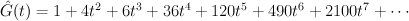$\hat{G}(t) = 1+4 t^2+6 t^3+36 t^4+120 t^5+490 t^6+2100 t^7+\cdots$

### The del Pezzo surface of degree 6

This is the blow-up of$\PP^2$ in three points. It is a toric variety. We have: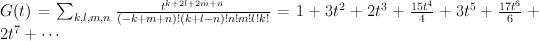$G(t) = \sum_{k,l,m,n} {t^{k+2l+2m+n} \over (-k+m+n)!(k+l-n)!n!m!l!k!} =1+3 t^2+2 t^3+\frac{15 t^4}{4}+3 t^5+\frac{17 t^6}{6}+2 t^7+\cdots$
and the regularized quantum period is: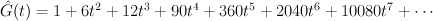$\hat{G}(t) = 1+6 t^2+12 t^3+90 t^4+360 t^5+2040 t^6+10080 t^7+\cdots$

### The del Pezzo surface of degree 5

This is a hypersurface of bidegree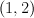$(1,2)$ in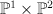$\PP^1 \times \PP^2$. We have: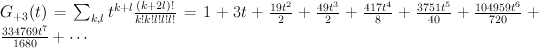$G_{+3}(t) = \sum_{k,l} t^{k+l} {(k+2l)! \over k!k!l!l!l!} = 1+3 t+\frac{19 t^2}{2}+\frac{49 t^3}{2}+\frac{417 t^4}{8}+\frac{3751 t^5}{40}+\frac{104959 t^6}{720}+\frac{334769 t^7}{1680}+\cdots$
and the regularized quantum period is: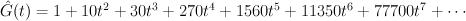$\hat{G}(t) = 1+10 t^2+30 t^3+270 t^4+1560 t^5+11350 t^6+77700 t^7+\cdots$

### The del Pezzo surface of degree 4

This is a (2,2) complete intersection in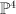$\PP^4$. We have: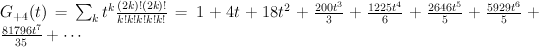$G_{+4}(t) = \sum_k t^{k} {(2k)!(2k)! \over k!k!k!k!k!} = 1+4 t+18 t^2+\frac{200 t^3}{3}+\frac{1225 t^4}{6}+\frac{2646 t^5}{5}+\frac{5929 t^6}{5}+\frac{81796 t^7}{35}+\cdots$
and the regularized quantum period is: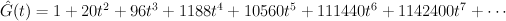$\hat{G}(t) = 1+20 t^2+96 t^3+1188 t^4+10560 t^5+111440 t^6+1142400 t^7+\cdots$

### The del Pezzo surface of degree 3

This is the cubic surface in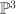$\PP^3$. We have: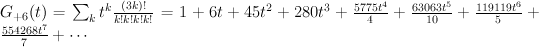$G_{+6}(t) = \sum_k t^{k} {(3k)!\over k!k!k!k!} = 1+6 t+45 t^2+280 t^3+\frac{5775 t^4}{4}+\frac{63063 t^5}{10}+\frac{119119 t^6}{5}+\frac{554268 t^7}{7}+\cdots$
and the regularized quantum period is: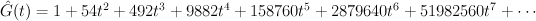$\hat{G}(t) = 1+54 t^2+492 t^3+9882 t^4+158760 t^5+2879640 t^6+51982560 t^7+\cdots$

### The del Pezzo surface of degree 2

This is the quartic surface in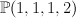$\PP(1,1,1,2)$. We have: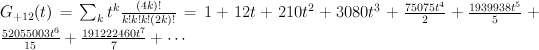$G_{+12}(t) = \sum_k t^{k} {(4k)!\over k!k!k!(2k)!} = 1+12 t+210 t^2+3080 t^3+\frac{75075 t^4}{2}+\frac{1939938 t^5}{5}+\frac{52055003 t^6}{15}+\frac{191222460 t^7}{7}+\cdots$
and the regularized quantum period is: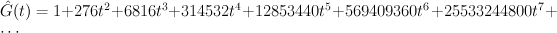$\hat{G}(t) = 1+276 t^2+6816 t^3+314532 t^4+12853440 t^5+569409360 t^6+25533244800 t^7 +\cdots$

### The del Pezzo surface of degree 1

This is the sextic surface in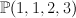$\PP(1,1,2,3)$. We have: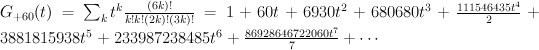$G_{+60}(t) = \sum_k t^{k} {(6k)!\over k!k!(2k)!(3k)!} = 1+60 t+6930 t^2+680680 t^3+\frac{111546435 t^4}{2}+3881815938 t^5+233987238485 t^6+\frac{86928646722060 t^7}{7}+\cdots$
and the regularized quantum period is: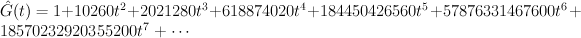$\hat{G}(t) = 1 + 10260 t^2 + 2021280 t^3 + 618874020 t^4 + 184450426560 t^5 + 57876331467600 t^6 + 18570232920355200 t^7+\cdots$

1.Sergey says:

And some mirror images:

# del Pezzo surfaces

Manifold Mirror image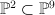$\PP^2 \subset \PP^9$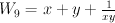$W_9 = x + y + \frac{1}{xy}$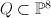$Q \subset \PP^8$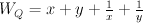$W_Q = x + y + \frac{1}{x} + \frac{1}{y}$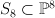$S_8 \subset \PP^8$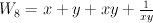$W_8 = x + y + xy + \frac{1}{xy}$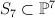$S_7 \subset \PP^7$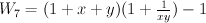$W_7 = (1 + x + y)(1 + \frac{1}{xy}) -1$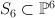$S_6 \subset \PP^6$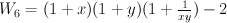$W_6 = (1+x)(1+y)(1+\frac{1}{xy}) -2$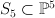$S_5 \subset \PP^5$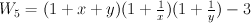$W_5 = (1+x+y)(1+\frac{1}{x})(1+\frac{1}{y}) -3$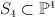$S_4 \subset \PP^4$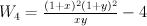$W_4 = \frac{(1+x)^2(1+y)^2}{xy} -4$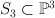$S_3 \subset \PP^3$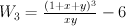$W_3 = \frac{(1+x+y)^3}{xy} -6$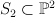$S_2 \subset \PP^2$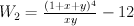$W_2 = \frac{(1+x+y)^4}{xy} -12$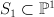$S_1 \subset \PP^1$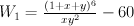$W_1 = \frac{(1+x+y)^6}{xy^2} -60$
2.Sergey says:

Shorter forms for non-normalized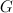$G$-series for del Pezzo surface of degree 6.

From description of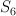$S_6$ as hyperplane section of Segre threefold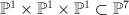$\PP^1 \times \PP^1 \times \PP^1 \subset \PP^7$: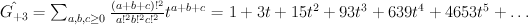$\hat{G_{+3}} = \sum_{a,b,c \geq 0} \frac{(a+b+c)!^2}{a!^2b!^2c!^2} t^{a+b+c} = 1 + 3 t + 15 t^2 + 93 t^3 + 639 t^4 + 4653 t^5 + \dots$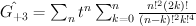$\hat{G_{+3}} = \sum_n t^n \sum_{k=0}^n \frac{n!^2 (2k)!}{(n-k)!^2 k!^4}$

From description of$S_6$ as linear section of Segre fourfold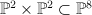$\PP^2 \times \PP^2 \subset \PP^8$: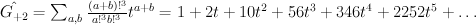$\hat{G_{+2}} = \sum_{a,b} \frac{(a+b)!^3}{a!^3 b!^3} t^{a+b} = 1 + 2 t + 10 t^2 + 56 t^3 + 346 t^4 + 2252 t^5 + \dots$

3.Sergey says:

Invariant T(X) (defined in these Split notes – Definition 28) for del Pezzo surfaces equals to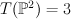$T(\PP^2) = 3$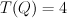$T(Q) = 4$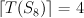$\lceil T(S_8) \rceil = 4$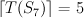$\lceil T(S_7) \rceil = 5$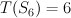$T(S_6) = 6$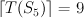$\lceil T(S_5) \rceil = 9$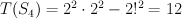$T(S_4) = 2^2 \cdot 2^2 - 2!^2 = 12$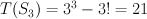$T(S_3) = 3^3 - 3! = 21$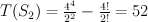$T(S_2) = \frac{4^4}{2^2} - \frac{4!}{2!} = 52$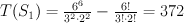$T(S_1) = \frac{6^6}{3^2 \cdot 2^2} - \frac{6!}{3! \cdot 2!} = 372$

Note that potentials I wrote down for del Pezzo surfaces of degrees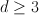$d\geq 3$ are _optimal_ – for them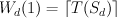$W_d(1) = \lceil T(S_d) \rceil$.
Potentials for del Pezzo surfaces of degrees 1 and 2 that I wrote down are not optimal: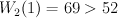$W_2(1) = 69 > 52$ and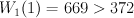$W_1(1) = 669 > 372$.
Nevertheless one can obtain from them the optimal potentials by doing a relatively short sequence of mutations (using this script).

4.Sergey says:

Can we beautify this page and stick it together with Fano 3-folds on top?

5.Tom says:

Done: see

for the new version, which should also appear in the menu at the top of the blog.